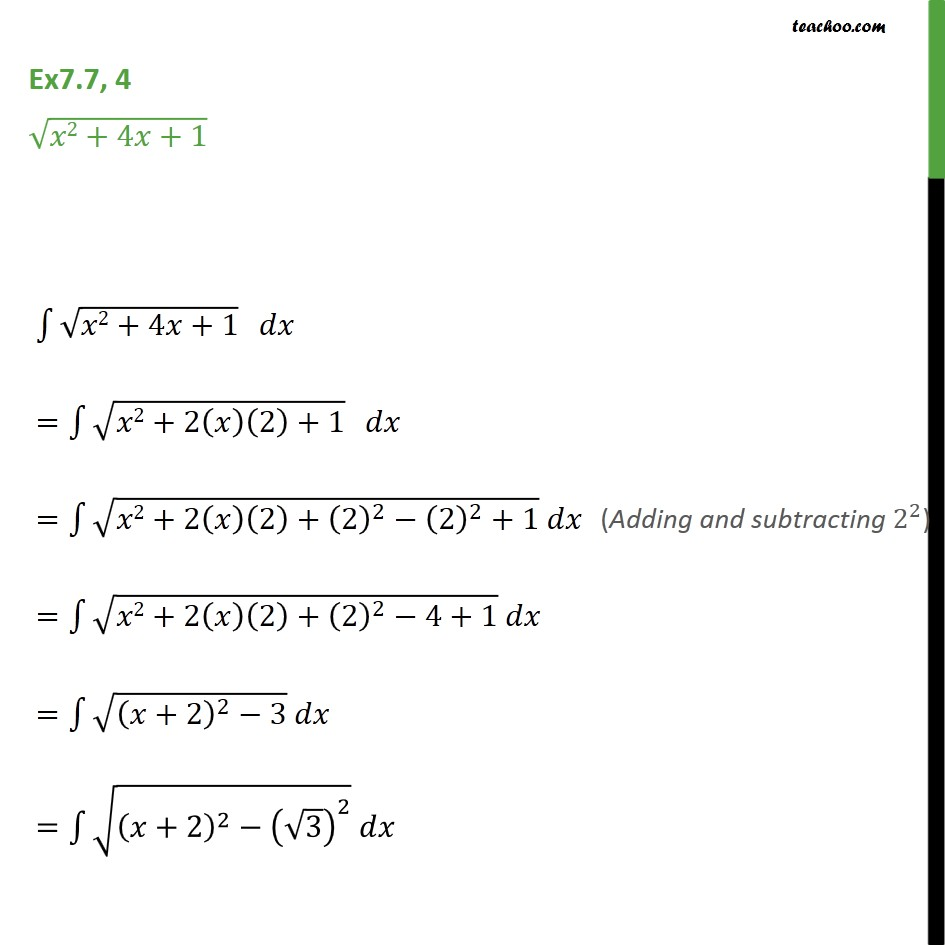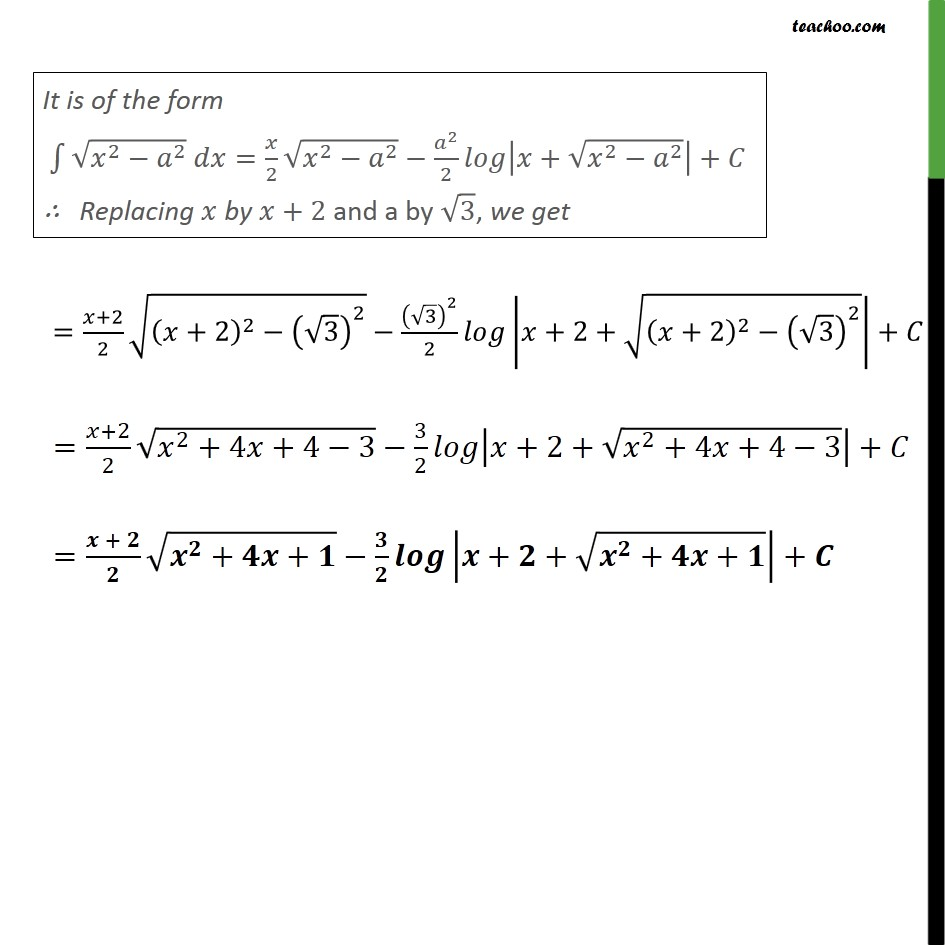Integration by specific formulaes - Formula 7

Chapter 7 Class 12 Integrals
Concept wiseLearn in your speed, with individual attention - Teachoo Maths 1-on-1 Class

### Transcript

Ex7.7, 4 2+4 +1 2+4 +1 = 2+2 2 +1 = 2+2 2 + 2 2 2 2 +1 = 2+2 2 + 2 2 4+1 = +2 2 3 = +2 2 3 2 = +2 2 +2 2 3 2 3 2 2 +2+ +2 2 3 2 + = +2 2 2 +4 +4 3 3 2 +2+ 2 +4 +4 3 + = + + + + + + + +